Courses

# Analog Electronics - 7

## 10 Questions MCQ Test RRB JE for Electronics & Communication Engineering | Analog Electronics - 7

Description
This mock test of Analog Electronics - 7 for Electronics and Communication Engineering (ECE) helps you for every Electronics and Communication Engineering (ECE) entrance exam. This contains 10 Multiple Choice Questions for Electronics and Communication Engineering (ECE) Analog Electronics - 7 (mcq) to study with solutions a complete question bank. The solved questions answers in this Analog Electronics - 7 quiz give you a good mix of easy questions and tough questions. Electronics and Communication Engineering (ECE) students definitely take this Analog Electronics - 7 exercise for a better result in the exam. You can find other Analog Electronics - 7 extra questions, long questions & short questions for Electronics and Communication Engineering (ECE) on EduRev as well by searching above.
QUESTION: 1

### For the Wien-bridge oscillator circuit shown below, the condition for bridge balance is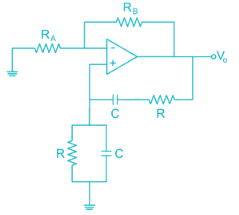Solution:

At oscillator frequency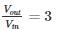i.e. gain of non-inverting amplifier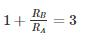⇒ RB = 2RA

QUESTION: 2

### Find the value of inductance in (mH) in the oscillator shown below to achieve a frequency of oscillation = 100 KHz is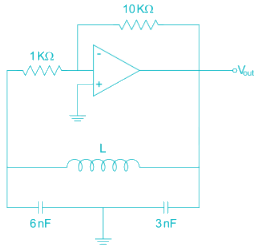Solution: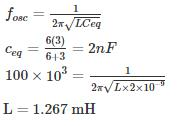*Answer can only contain numeric values
QUESTION: 3

### For the circuit shown in the figure the value of R to get oscillation of 100 kHz is ________ KΩ.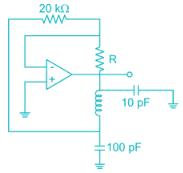Solution:

The given oscillator is Colpitts’s oscillator

The condition for oscillation is

|Aβ|=1

A = -R2/R1

β=C2/C1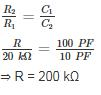*Answer can only contain numeric values
QUESTION: 4

The figure shows a phase-shift oscillator circuit with voltage follower buffer stages.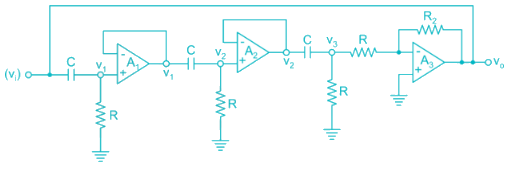The value of R2/R required for sustained oscillations is _____.

Solution:
• The basic amplifier of the circuit is the op-amp A3.
• The output of amplifier is connected to 3 stage RC filter.

Transfer function of first RC network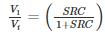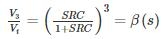The amplifier A3 is connected in inverting mode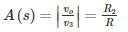The loop gain is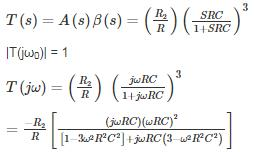Substitute real part of denominator to zero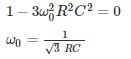At this frequency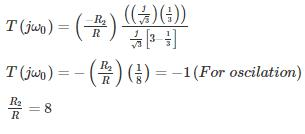QUESTION: 5

The value of R in oscillator circuit shown in a given circuit below is chosen such that it oscillates at an angular frequency of ω. The value of ω and the required value of R will respectively be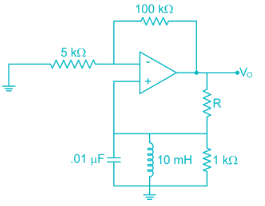Solution:

Hartley oscillator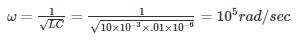Amp with +ve F.B

Loop again AB = 1 + j0

At oscillation

∠AB = 0 (no reactance XL = XC)

At frequency of oscillation look lies resistive Network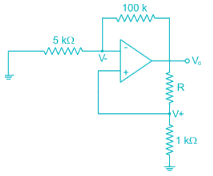For ideal op amp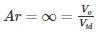Use voltage division Rule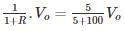R + 1 = 21

R = 20 kΩ

R = 2 × 104Ω

*Answer can only contain numeric values
QUESTION: 6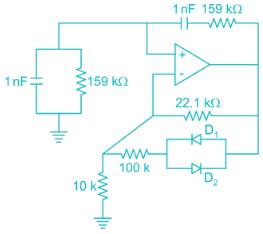The frequency of oscillation of the circuit in kilohertz is ______ kHz.

Solution:

The given circuit is wein bridge oscillator.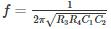R3 = R4 = 159 KΩ

C1 = C2 = 1 nF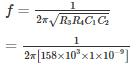= 103 HZ

= 1 KHZ

QUESTION: 7

For the transistor oscillator circuit given below the frequency of oscillation is 8.5 kHz. The value of the collector resistor approximately is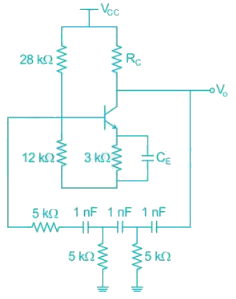Solution:

In a transistor phase shift oscillator the oscillation frequency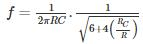R, C are the resistor and the capacitor in phase shift circuit

RC is collector capacitance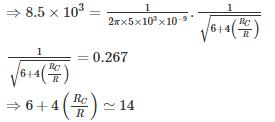⇒ RC = 2R = 10 kΩ

*Answer can only contain numeric values
QUESTION: 8

The oscillation frequency if the below-shown oscillator is 1 kHz then the value of ‘L’ is ______ mH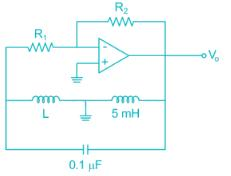Solution:

The given oscillator is Hartley oscillator, where the oscillation frequency.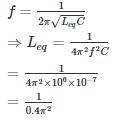= 253.3 mH

Leq = L + 5 mA

⇒ L = 253.3 – 5

= 248. 3 mH

QUESTION: 9

For the circuit shown the value of R1 and R2 such that the circuit generates oscillations of frequency 274 Hz is [Assume the time constant of the feedback RC network is 0.01 sec]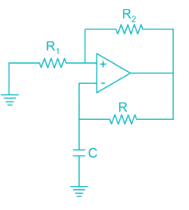Solution:

Given figure is of A-stable multi-vibrator,

Time constant is given by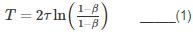β = feedback factor

τ = time constant of feedback loop: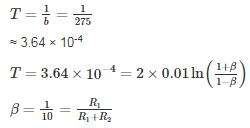Option 3 satisfies the condition

*Answer can only contain numeric values
QUESTION: 10

The frequency of oscillation of the circuit shown below is ________ Hz.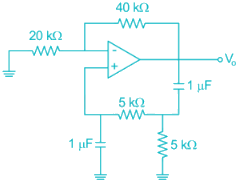Solution:

The given oscillator is Wien-bridge oscillator with R = 5 kΩ, C = 1 μF

⇒ frequency of oscillation f = 1/2πRC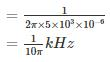= 31.8 Hz